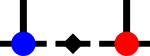SyTentemplate<typename X , typename FunctionEval , typename GradientEval , typename LinesearchEval , typename RandomStep , typename Terminator >
 auto syten::GradientOpt::gradient_descent_linesearch ( X x, FunctionEval func, GradientEval grad, LinesearchEval lin, RandomStep rand, Terminator terminate, Index num_steps = 100, Index ls_num_steps = 50, SRDef ls_lower = 0, SRDef ls_upper = 1 )

Attempt at a gradient descent with linesearch.

 X domain type FunctionEval type of the function evaluation function, taking an X GradientEval type of the gradient evaluation function, taking a result of the function and its X LinesearchEval type of the linesearch evaluation function, taking a step size a, the current iteration point x and the gradient g and returning the value of f(x-a*g) and f'(x-a*g) (wrt a). RandomStep type of the random stepper to be enacted if nothing else works. Takes the step size (either 0 or NAN) point and the gradient. Terminator type of the termination condition, returning true if we should break out. Takes the step size, current function value and current gradient.Here is the call graph for this function:Here is the caller graph for this function: